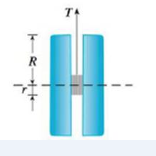Chapter 14.4, Problem 37E

Chapter
Section
Textbook Problem

The tension T in the string of the yo-yo in the figure is T = m g R 2 r 2 + R 2 where m is the mass of the yo-yo and g is acceleration due to gravity. Use differentials to estimate the change in the tension if R is increased from 3 cm to 3.1 cm and r is increased from 0.7 cm to 0.8 cm. Does the tension increase or decrease?To determine

To estimate: The change in the tension T of the yo-yo by using differentials; infer whether the tension increase or decrease.

Explanation

Given:

The tension T in the string of the yo-yo is T=mgR2r2+R2 , where m is the mass of yo-yo, g is acceleration due to gravity, R is outer radius, r is inner radius.

The outer radius R=3cm .

The inner radius r=0.7cm .

The error in outer radius dR=0.1cm .

The error ininner radius dr=0.1cm .

Calculation:

Acoording to the given data outer radius and inner radius (R,r) change from (3,0.7) to (3.1,0.8) .

The change in the tension of yo-yo is calculated by, dT=TRdR+Trdr .

Take partial derivative of T with respect to R ,

TR=(2r2+R2)(mg)(1)mgR(2R)(2r2+R2)2[R(uv)=u'vv'uv2]=mg[2r2+R22R2](2r2+R2)2=mg(2r2R2)(2r2+R2)2

Take partial derivative of T with respect to r ,

TR=(2r2+R2)(0)mgR(2(2r))(2r2+R2)2

Still sussing out bartleby?

Check out a sample textbook solution.

See a sample solution

The Solution to Your Study Problems

Bartleby provides explanations to thousands of textbook problems written by our experts, many with advanced degrees!

Get Started